# 圆上任意角度的点的坐标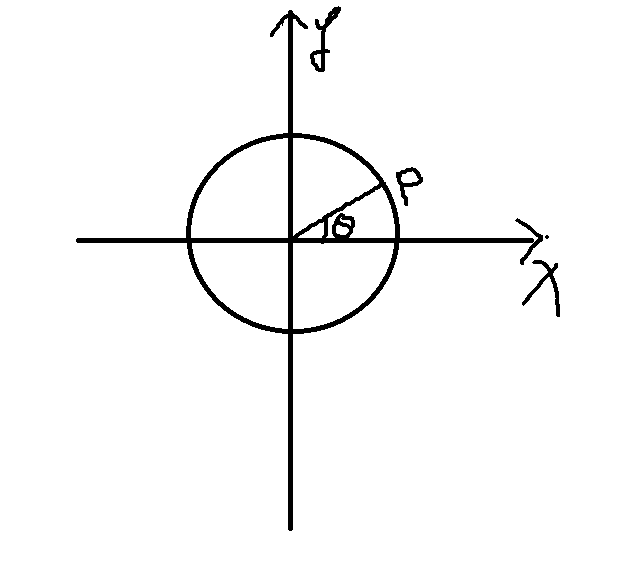$\left\{\begin{matrix} px= Cx+Rcos(\theta) \\ py= Cy+Rsin(\theta) \end{matrix}\right.$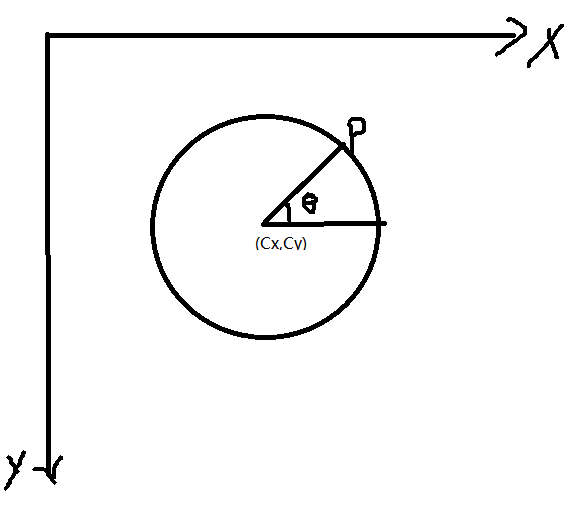$\left\{\begin{matrix} px= Cx+Rcos(\theta) \\ py= Cy-Rsin(\theta) \end{matrix}\right.$

# 椭圆上任意角度的点的坐标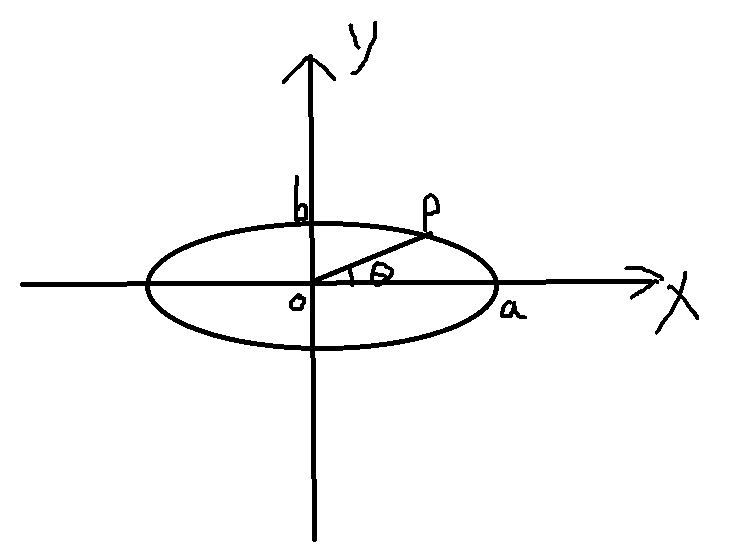$\left\{\begin{matrix} \frac{x^2}{a^2}+\frac{y^2}{b^2}&=&1 \\ \frac{y}{x}& =& \tan(\theta) \end{matrix}\right.$

$x^2=\frac{a^2b^2}{b^2+a^2\tan^2(\theta)}$

(一). $0\leq\theta<pi/2$ 或者 $\frac{3*pi}{2}<\theta \leq 2*pi$
$\left\{\begin{matrix} x=\frac{ab}{\sqrt{b^2+a^2\tan^2(\theta)}} \\ y=\frac{ab\tan(\theta)}{\sqrt{b^2+a^2\tan^2(\theta)}} \end{matrix}\right.$
(二). $pi/2<\theta<\frac{3*pi}{2}$
$\left\{\begin{matrix} x=-\frac{ab}{\sqrt{b^2+a^2\tan^2(\theta)}} \\ y=-\frac{ab\tan(\theta)}{\sqrt{b^2+a^2\tan^2(\theta)}} \end{matrix}\right.$
(三). $\theta = pi/2$
$\left\{\begin{matrix} x=0 \\ y=b \end{matrix}\right.$
(四). $\theta = \frac{3*pi}{2}$
$\left\{\begin{matrix} x=0 \\ y=-b \end{matrix}\right.$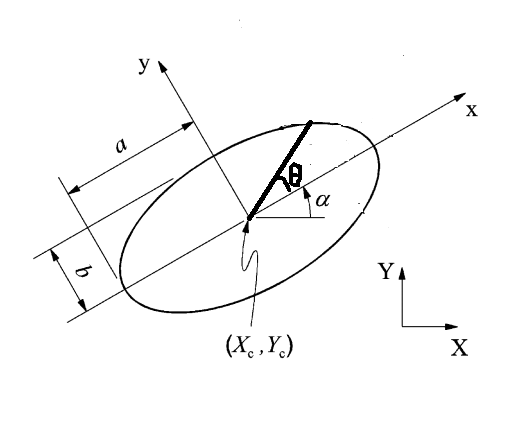$\begin{pmatrix} x\\ y \end{pmatrix}=R(\begin{pmatrix} X\\ Y \end{pmatrix}-\begin{pmatrix} X_c\\Y_c \end{pmatrix})$

$R=\begin{pmatrix} cos(-\alpha) &-sin(-\alpha) \\ sin(-\alpha) & cos(-\alpha) \end{pmatrix}=\begin{pmatrix} cos(\alpha) &sin(\alpha) \\ -sin(\alpha) & cos(\alpha) \end{pmatrix}$

$\begin{pmatrix} X\\ Y \end{pmatrix} =R^{-1}\begin{pmatrix} x\\ y \end{pmatrix}+\begin{pmatrix} X_c\\Y_c \end{pmatrix}$

$R^{-1}=R^{T}=\begin{pmatrix} cos(\alpha) &-sin(\alpha) \\ sin(\alpha) & cos(\alpha) \end{pmatrix}~~~~~~~~~~~~~~~~~~~~~~~~~~~(\bigstar)$

1. 如果以上的角度是相对于水平轴的角度，则对应的椭圆上的点的坐标如何求呢？
答： 其实很简单，只需要：
$\theta'=\left\{\begin{matrix} \theta-\alpha+2*pi, & \theta<\alpha \\ \theta-\alpha, & \theta \geq \alpha \end{matrix}\right.$
$\theta'$替换以上标准椭圆下的$\theta$即可。
2. 如果竖直坐标轴为竖直向下的，即为图像坐标系下的椭圆，那么如何求对应的角度？
答：
需要做两方面的改变即可：
$\left\{\begin{matrix} \alpha\rightarrow -\alpha\\ y\rightarrow -y \end{matrix}\right.$
第一行改变$\bigstar$出的$\alpha$. 第二行改变标准椭圆下的y值的符号。

# matlab代码

demo.m
################################
center_x=282;
center_y=263;
phi=pi/6;
R1=141;
R2=62;
%DrawEllipse([center_x,center_y],R1,R2,phi);
axis equal;
hold on;
set(gca,'ydir','reverse')
for angle=linspace(0,3*pi/2,360)
[ px,py ] = get_points_ellipse(center_x, center_y,phi,R1,R2, angle );
plot(px,py,'ro');
hold on;
end
axis([0,500,0,500])

function [ px,py ] = get_points_ellipse(center_x, center_y,phi,R1,R2, angle )
% 求椭圆某个角度上的点的坐标。
%https://math.stackexchange.com/questions/22064/calculating-a-point-that-lies-on-an-ellipse-given-an-angle
%https://blog.csdn.net/xiamentingtao/article/details/54934467

%https://stackoverflow.com/questions/17762077/how-to-find-the-point-on-ellipse-given-the-angle
%另一种解法  从极坐标的角度出发。

% 椭圆参数  圆心 (center_x.center_y) 角度 phi(-pi,pi)，实际上仅考虑（0，pi） 沿着角度的主轴半径R1，另一主轴对应的半径R2
% 求相对于水平轴x轴上angle（基于0-2pi之间）上对应的点坐标。
% 注意求解的坐标系为：水平向右为x轴,竖直向上为y轴。 如果竖直向下为y轴，则下面的y应该变换成-y， phi变成-phi

%先将斜的椭圆转正，并平移到原点。方法就是平移到中心，且旋转-phi角度,再应用标准椭圆求取点。
if(angle<phi)
theta = angle-phi+2*pi;  %想对于主轴的角度
else
theta = angle-phi;
end
% theta为相对于主轴的角度
alpha = -phi;    %与竖直的轴有关，如果是竖直向上，则变为正phi
% 先计算标准椭圆 x^2/R1^2+y^2/R2^2=1  与 直线 y/x=tan(angle)的交点。
assert(angle>=0 && angle<=2*pi);
if(theta==pi/2)
x=0;
y=R2;
elseif(theta==pi*3/2)
x=0;
y=-R2;
elseif(theta>pi/2 && theta<pi*3/2)
x=-R1*R2/sqrt(R2^2+R1^2*tan(theta)^2);
y=-R1*R2*tan(theta)/sqrt(R2^2+R1^2*tan(theta)^2);
else
x=R1*R2/sqrt(R2^2+R1^2*tan(theta)^2);
y=R1*R2*tan(theta)/sqrt(R2^2+R1^2*tan(theta)^2);
end
y=-y;    %与竖直的轴有关，如果是竖直向上，则变为正y
% 将以上结果转换回去
px= cos(alpha)*x-sin(alpha)*y+center_x;
py=sin(alpha)*x+cos(alpha)*y+center_y;

end


function DrawEllipse(C,a,b,alpha)
% DRAWELLIPSE plots an ellipse
%   DrawEllipse(C,a,b,alpha) plots ellipse with center C, semiaxis a
%   and b and angle alpha between a and the x-axis

s=sin(alpha); c=cos(alpha);
Q =[c -s; s c]; theta=[0:0.02:2*pi];
u=diag(C)*ones(2,length(theta)) + Q*[a*cos(theta); b*sin(theta)];
plot(u(1,:),u(2,:));
hold on;
plot(C(1),C(2),'+');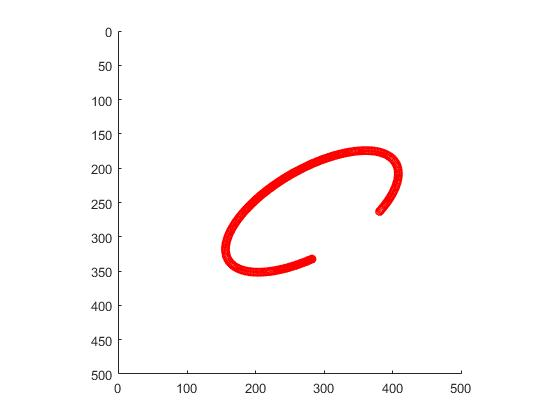# 另一种基于极坐标变换的高效实现【转载】

CDoublePoint2d GetPointOnEllipse(const CDoublePoint2d& ptCenter, double a, double b, double radian, double dChangZhouAngle)
{
double x = a*cos(dLiXin)cos(dChangZhouAngle) - bsin(dLiXin)sin(dChangZhouAngle) + ptCenter.x;
double y = a
cos(dLiXin)sin(dChangZhouAngle) + bsin(dLiXin)*cos(dChangZhouAngle) + ptCenter.y;
return CDoublePoint2d(x, y);
}

# 参考文献